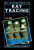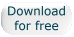Errata for first printing of "An Introduction to Ray Tracing"Last changed: April 15, 2019An Introduction to Ray Tracing, edited by Andrew Glassner, Morgan Kaufmann, 1989. The first book on ray tracing. Ancient, but most of the information is still valid - math is math, data structures are data structures. Download the PDF (recommended) or DJVU version for free. Find other ray tracing books, free and otherwise, on our Ray Tracing Resources Page. Errata The errata that follows are errors reported since January 20, 2019, when the book was released as a free PDF and DJVU file. These errors are corrected in the free PDF version of the book (but not the DJVU): p. 138-141, sections 2.6 and 2.7, have been rewritten entirely, due to a cascading effect of small algebraic blunders near the start. These are in the PDF as pages 138-rev1 and 139-rev1. (superceded by the fix above, but one error in the original text that is fixed in the PDF) p. 139, in the third line of equation (7c), the "T" should be "N" (since going from the second to third line is substituting in the relationship T = \alpha I + \beta N from eq (6b). (thanks to Matt Pharr) p. 327, "Path racing" should be "Path tracing". (thanks to Matt Pharr) The remaining errata below is originally from The Wayback Machine. All of these errors are corrected in the PDF and DJVU versions. This errata listing is here for people who have the first printing of the book. p. 86, last paragraph before Algebraic Surfaces header: change "where all the the intersections of the ray with all the objects in the CSG tree are required" to end as "may be required". ----- p. 88, equation "t = etc": change from "t = etc" to "t = -etc", i.e. a minus sign is missing, so negate the right hand side of the equation. ----- p. 91, last formula in the Sphere section (in the text): change to x1^2 + y1^2 + z1^2 = 1 ^ subscript was "0" ----- p. 91, in the Paraboloid section, second equation: change the two minus signs to plus signs (i.e. before z1 and z0). ----- p. 93, torus equation corrections: change two equations a2 = 2(x1^2+y1^2+z1^2)((x0^2+y0^2+z0^2)-(a^2+b^2)) + 4 * (x0x1+y0y1+z0z1)^2 + 4a^2z1^2 ^ ^^ ^ was "2" add was "-" a1 = 4 * (x0x1+y0y1+z0z1)((x0^2+y0^2+z0^2)-(a^2+b^2)) + 8a^2 * z0 * z1 ^ was "-" a0 = ((x0^2+y0^2+z0^2)-(a^2+b^2))^2 - 4a^2(b^2-z0^2) ^ squaring was left off ----- p. 95, last sentence before the Simplicial Splines and Steiner Patches section: change "of" to "or, i.e. it should read "numerical techniques or subdivision algorithms". ----- p. 95, last formula on the page: change to z(u,v) = h y(v) ^ subscript was "x" ----- p. 100, Figure 7 is wrong. The upper figure is in error; the lower part is correct. In the upper figure, the outer contour is the silhouette of a 3D parametric surface. Curve c1 is the intersection of one plane with that surface, and c2 is the intersection of another plane, perpendicular to the first. The line of intersection of the two planes is collinear with the ray, indicated by the line with the arrow. The other three lines in the figure are extraneous and should be ignored. The lower part of the figure shows the two curves in uv space. ----- p. 101, the last sentence in the Bicubic Patches section: change "this can involve a loss of extra computation" should be "this can involve extra computation". ----- p. 101, the second equation in the Numerical Methods section: change the second "=" in the line to a "+" (it's the only equation with two "=" in it). ----- p. 105, the 2-D line equation: change to (y1)x - (x1)y - (x0y1 - y0x1) = 0 ^ was a "+" ----- p. 108, formula for f: This does not agree with Fig. 10. Using the same notation as in the figure, change to: For this shape, f is 2 2 2 2 (x-r (u)) + (y-r (u)) + (z - r (u)) - a (u) = 0 x y z where (r , r , r ) is the center of the sphere and a is the radius. x y z ----- p. 140, equation (7h): missing right parenthesis, change to "...- 1)))N." ----- p. 148, section on distribution term D, 8th line: change "the angle between L and H" to "the angle between N and H". ----- p. 156, section 5.4, 2nd paragraph: change "spectral transmission curve" to "specular transmission curve". ----- p. 158, in Fdt(lambda) definition: change "diffuse reflection" to "diffuse transmission". ----- p. 158, line immediately after Fdt(lambda) definition: change "We note that the diffuse reflectance" to "We note that the diffuse transmittance". ----- p. 238, Fig. 24: A chunk is missing in the upper left corner. The labels should read: "Directions crossed with", and "Applies to". ----- p. 260, reference by Gervautz: change "Comput. Graph." to "Computers and Graphics". ----- p. 288, immediately after the DERIVATION OF REFRACTION FORMULAS header: The introductory paragraph is missing. It reads: We derive three alternative formulas for the refracted ray direction in ray tracing in order to prove their equivalence and to demonstrate the process of translating physical laws into optimized computational formulas. It is common knowledge that light rays refract when they strike an interface between two different transparent media, such as air-water, air-glass, or glass-water. In 1621 Dutch mathematician Willebrord Snell discovered a formula quantifying this observation: the ratio of the sines of the incident and refracted angles equals the ratio of the indices of refraction of the two materials. Snell's law is: eta sin( theta ) = eta sin( theta ) 1 1 2 2 where theta-sub-1 is the angle of incidence, theta-sub-2 is the angle of refraction (both measured from the perpendicular to the interface) and eta-sub-1 and eta-sub-2 are the two indices of refraction on the incident and refracted sides of the interface, respectively. Light passing through a material slows relative to its speed in a vacuum by a factor equal to the index of refraction of that material. In fact, Snell's law is a simple consequence of this speed variation and Fermat's _Principle of Least Time_, which states that light takes the fastest path to get from one point to another [Feynman63]. For computation we need to recast Snell's law in terms of (x,y,z) direction vectors. This can be done in several different ways. In the derivations below we make extensive use of angles and trigonometry, but thankfully, it is possible to eliminate all of these terms from the final formulas, so theta-sub-1 and theta-sub-2 need never be computed. As a convention, vectors are upper case and scalars are lower case. ----- END